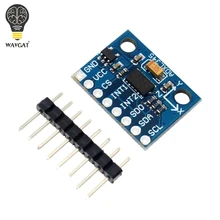In this example we show how to connect a ADXL345 3-axis accelerometer to a MSP-EXP432P401R LaunchPad, the example will use the Energia IDE.

I used a ADXL345 module in this example, which you can see belowThe ADXL345 is a small, thin, low power, 3-axis accelerometer with high resolution (13-bit) measurement at up to ±16g. Digital output data is formatted as 16-bit twos complement and is accessible through either a SPI (3- or 4-wire) or I2C digital interface.

The ADXL345 is well suited for mobile device applications. It measures the static acceleration of gravity in tilt-sensing applications, as well as dynamic acceleration resulting from motion or shock. Its high resolution (4 mg/LSB) enables measurement of inclination changes less than 1.0°.

Connection

Here is a picture of the launchpad so you can see what pins we are referring to below in the table

 Module Connection MSP432 Connection SDA J1-10 SDA SCL J1-9 SCL Gnd J3-22 Gnd Vcc J1-1 3.3v

Code

 Source code```#include <Wire.h>

void setup()
{
// Initialise I2C communication as MASTER
Wire.begin();
// Initialise serial communication, set baud rate = 9600
Serial.begin(9600);

// Start I2C Transmission
// Select bandwidth rate register
Wire.write(0x2C);
// Normal mode, Output data rate = 100 Hz
Wire.write(0x0A);
// Stop I2C transmission
Wire.endTransmission();

// Start I2C Transmission
// Select power control register
Wire.write(0x2D);
// Auto-sleep disable
Wire.write(0x08);
// Stop I2C transmission
Wire.endTransmission();

// Start I2C Transmission
// Select data format register
Wire.write(0x31);
// Self test disabled, 4-wire interface, Full resolution, Range = +/-2g
Wire.write(0x08);
// Stop I2C transmission
Wire.endTransmission();
delay(300);
}

void loop()
{
unsigned int data;
for(int i = 0; i < 6; i++)
{
// Start I2C Transmission
// Select data register
Wire.write((50 + i));
// Stop I2C transmission
Wire.endTransmission();

// Request 1 byte of data

// Read 6 bytes of data
// xAccl lsb, xAccl msb, yAccl lsb, yAccl msb, zAccl lsb, zAccl msb
if(Wire.available() == 1)
{
}
}

// Convert the data to 10-bits
int xAccl = (((data & 0x03) * 256) + data);
if(xAccl > 511)
{
xAccl -= 1024;
}
int yAccl = (((data & 0x03) * 256) + data);
if(yAccl > 511)
{
yAccl -= 1024;
}
int zAccl = (((data & 0x03) * 256) + data);
if(zAccl > 511)
{
zAccl -= 1024;
}

// Output data to serial monitor
Serial.print("Acceleration in X-Axis is : ");
Serial.println(xAccl);
Serial.print("Acceleration in Y-Axis is : ");
Serial.println(yAccl);
Serial.print("Acceleration in Z-Axis is : ");
Serial.println(zAccl);
delay(300);
}```

Output

Open the serial monitor and you should see something like this

Acceleration in X-Axis is : 141
Acceleration in Y-Axis is : -74
Acceleration in Z-Axis is : 200
Acceleration in X-Axis is : 142
Acceleration in Y-Axis is : -74
Acceleration in Z-Axis is : 199
Acceleration in X-Axis is : 141
Acceleration in Y-Axis is : -74
Acceleration in Z-Axis is : 199
Acceleration in X-Axis is : 129
Acceleration in Y-Axis is : -59
Acceleration in Z-Axis is : 272
Acceleration in X-Axis is : 203
Acceleration in Y-Axis is : -72
Acceleration in Z-Axis is : 160